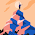## Sunday, December 2, 2012

### Numeric CAS - Part 3: Synthetic Division

Synthetic Division

Goal: Divide the polynomial p(x) = a_n x^n + a_(n-1) x^(n-1) + ... + a_0 by (x - r).

p(x) ÷ (x - r) = q_n x^n + q_(n-1) x^(n-1) + ... + q_0 with remainder E

Input: A list of coefficients {a_n, a_(n-1), a_(n-2), ... , a_0} (List 1)

Output: Resulting coefficients {q_n, q_(n-1), ... , q_0, E} (List 2)

Example: (2x^3 + x - 3) ÷ (x - 3) = 2x^2 + 6x + 19 + 54 ÷ (x - 3)

List 1: {2, 0, 1, -3}, R = 3
List 2: {2, 6, 19, 54}

Casio Prizm

POLYSYN
Synthetic Division - 160 bytes

"P(X) ÷ (X-R)"
"{AnX^n,...,A0}"
"LIST:"?→List 1
"R"?→R
List 1 → List 2
For 1→K To Dim List 1 - 1
R × List 2[K] + List 1[K+1] → List 2[K+1]
Next
"LAST TERM = REMAINDER" ◢
List 2

TI-84+

POLYSYN
Synthetic Division - 143 bytes

: Disp "P(X)/(X-R)"
: Disp "{AnX^n,...,A0}"
: Input "LIST:", L1
: Input "R:", R
: L1->L2
: For(K,1,dim(L1)-1)
: R*L2(K)+L1(K+1)->L2(K+1)
: End
: Disp "LAST TERM=", "REMAINDER"
: Pause L2

HP 39gii

POLYSYN
Synthetic Division
11/23/2012

L1 and A are prompted. The resulting is a list of coefficients, with the last term the remainder

Example: (21x^2 + 42x + 144)/(x - 12) = 21x + 294 + 3672/(x-12)
Output List: {21, 294, 3672}

EXPORT POLYSYN()
BEGIN
LOCAL K,S,T;
EDITLIST(L1);
INPUT(R,"P(X)/(X-R)");
L1 → L2;
SIZE(L1)→ S;
S-1 → T;
FOR K FROM 1 TO T DO
R * L2(K) + L1(K+1) → L2(K+1);
END;
MSGBOX("LAST TERM=REMAINDER");
RETURN L2;
END;

This blog is property of Edward Shore. 2012

#### 2 comments:

1.this for creating an app or what. seems interesting this codes for solving the synthetic division

2.this for creating an app or what. seems interesting this codes for solving the synthetic division

### HP Prime and HP 41C/DM 41L: Sum of Two Squares

HP Prime and HP 41C/DM 41L:  Sum of Two Squares  Introduction Given a positive integer n, can we find two non-negative integers x and y...